Evaluation Hardware \$225.00••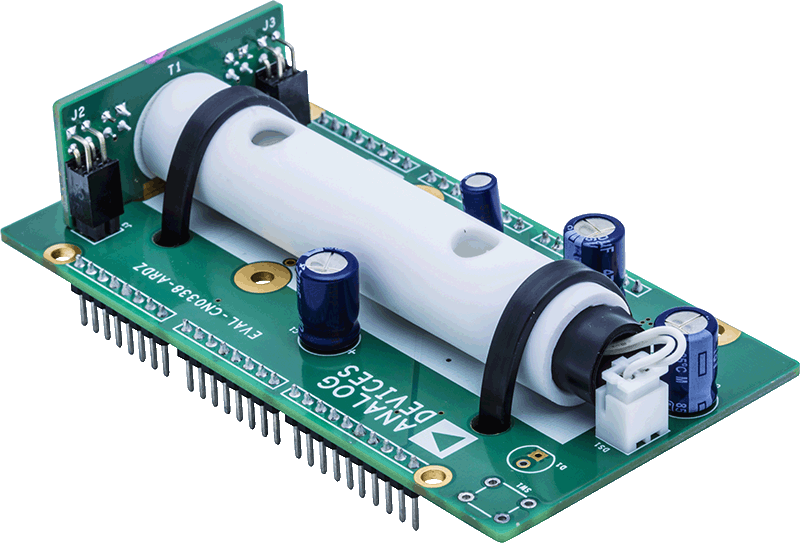••# Overview

## Design Resources

### Design & Integration Files

• Schematic
• Bill of Materials
• Gerber Files
• Layout Files (Allegro)

### Evaluation Hardware

Part Numbers with "Z" indicate RoHS Compliance. Boards checked are needed to evaluate this circuit

• EVAL-CN0338-ARDZ (\$225.00) NDIR Carbon Dioxide Gas Detector

## Features & Benefits

• Gas sensor based on NDIR
• Optimized for CO2
• Arduino shield form factor for rapid prototyping

# Circuit Function & Benefits

The circuit shown in Figure 1 is a complete thermopile-based gas sensor using the nondispersive infrared (NDIR) principle. This circuit is optimized for CO2 sensing, but can also accurately measure the concentration of a large number of gases by using thermopiles with different optical filters.Figure 1. NDIR Gas Sensing Circuit (Simplified Schematic: All Connections and Decoupling Not Shown)

The printed circuit board (PCB) is designed in an Arduino shield form factor and interfaces to the EVAL-ADICUP360 Arduino-compatible platform board. The signal conditioning is implemented with the AD8629 and the ADA4528-1 low noise amplifiers and the ADuCM360 precision analog microcontroller, which contains programmable gain amplifiers, dual 24-bit Σ-Δ analog-to-digital converters (ADCs), and an ARM Cortex-M3 processor.

# Circuit Description

The thermopile sensor is composed of a large number of thermocouples connected usually in series or, less commonly, in parallel. The output voltage of the series-connected thermocouples depends on the temperature difference between the thermocouple junctions and the reference junctions. This principle is called the Seebeck effect.

The circuit uses the AD8629 op amp to amplify the thermopile sensor output signals. The relatively small output voltage of the thermopile (from hundreds of microvolts to several millivolts) requires high gain with very low offset and drift to avoid dc errors. The high impedance (typically 84 kΩ) of the thermopile requires low input bias current to minimize errors, and the AD8629 bias current is only 30 pA typical. The very low drift with time and temperature eliminates additional errors once the temperature measurement has been calibrated. A pulsed light source synchronized with the ADC sampling rate minimizes the errors caused by low frequency drift and flicker noise.

The AD8629 only has 22 nV/√Hz voltage noise spectral density at 1 kHz, which is less than the thermopile voltage noise density of 37 nV/√Hz.

The AD8629 also has a very low current noise spectral density of 5 fA/√Hz typical at 10 Hz. This current noise flows through the 84 kΩ thermopile and only contributes 420 pV/√Hz at 10 Hz.

With the 200 mV common-mode voltage buffered by low noise amplifier ADA4528-1, the NTC and thermopile signal output meets the requirements of the ADuCM360 buffered mode input. AGND + 0.1V to approximately AVDD − 0.1 V for ADuCM360 ADC buffered mode input. The CN-0338 Arduino shield board can be compatible with other types of Arduino-compatible platform board with single-input ADCs only.

The circuit chopping frequency can be 0.1 Hz to 5 Hz selected by software. The ADP7105 low dropout regulator generates a stable 5 V output voltage to drive the lamp, and is turned on and off by the ADuCM360. The soft start feature of the ADP7105 eliminates the in-rush current when cold-starting the lamp.

The ADuCM360 includes dual, 24-bit, Σ-Δ ADCs for simultaneous sampling of a dual element thermopile at programmable rates of 3.5 Hz to 3.906 kHz. The data rate in the NDIR system is limited from 3.5 Hz to 483 Hz for best noise performance.

Theory of Operation

To understand the thermopile, it is useful to review the basic theory of thermocouples. If two dissimilar metals are joined at any temperature above absolute zero, there is a potential difference between them (their thermoelectric EMF or contact potential), which is a function of the temperature of the junction (see the thermoelectric EMF circuit in Figure 2). This effect is called the Seebeck effect after its discoverer, Thomas Johann Seebeck.Figure 2. Thermocouple Principles

If the two wires are joined at two places, two junctions are formed (see the thermocouple connected to load in Figure 2). If the two junctions are at different temperatures, there is a net EMF in the circuit, and a current flows determined by the EMF and the total resistance in the circuit (see Figure 2). If one of the wires is broken, the voltage across the break is equal to the net thermoelectric EMF of the circuit, and if this voltage is measured, it can be used to calculate the temperature difference between the two junctions (see the thermocouple voltage measurement in Figure 2). Remember that a thermocouple measures the temperature difference between two junctions, not the absolute temperature at one junction. The temperature at the measuring junction be measured only if the temperature of the other junction (often called the reference junction or the cold junction) is known.

However, it is not so easy to measure the voltage generated by a thermocouple. Suppose that a voltmeter is attached to the first thermocouple measurement circuit (see the practical thermocouple voltage measurement showing cold junction in Figure 2). The wires attached to the voltmeter form further thermocouples where they are attached. If both these additional junctions are at the same temperature (it does not matter what temperature), the Law of Intermediate Metals states that they make no net contribution to the total EMF of the system. If they are at different temperatures, they introduce errors. Because every pair of dissimilar metals in contact generates a thermoelectric EMF—including copper/solder, kovar/copper (kovar is the alloy used for IC leadframes) and aluminum/kovar (at the bond inside the IC)—the problem is even more complex in practical circuits, and it is necessary to take extreme care to ensure that all the junction pairs in the circuitry around a thermocouple, except for the measurement and reference junctions themselves, are at the same temperature.

A thermopile consists of a series connection of a large number of thermocouples, as shown in Figure 3. Compared to a single thermocouple, the thermopile generates much higher thermoelectric voltage.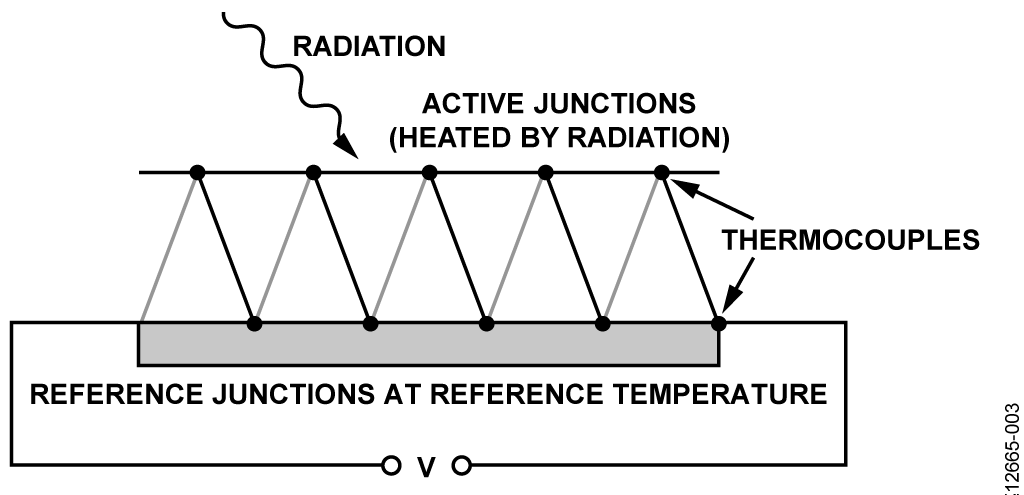Figure 3. Thermopile Constructed of Multiple Thermocouples

In the NDIR application, pulsed and filtered IR light is applied to the series-connected active junctions; the junctions are therefore heated, which in turn generates a small thermoelectric voltage. The temperature of the reference junction is measured with a thermistor.

Many gases have permanently or nonpermanently separated centers of positive and negative charge. The gases are able to absorb specific frequencies in the infrared spectrum, which can be used for gas analysis. When infrared radiation is incident on the gas, the energy states of atoms vibrating in the molecules change in discrete steps when the wavelength of the infrared matches the molecules natural frequencies or resonances.

For a majority of IR gas sensing applications, the identities of the target gases are known; therefore, there is little need for gas spectrometry. However, the application must deal with a certain amount of cross sensitivity between different gases if their absorption lines overlap.

Carbon dioxide has a very strong absorption band between 4200 nm and 4320 nm, as shown in Figure 4.Figure 4. Absorption Spectrum of Carbon Dioxide (CO2)

The available output range of IR sources and the absorption spectrum of water also govern the choice of the sensing wavelengths. Water shows strong absorptions below 3000 nm and also between 4500 nm and 8000 nm. Any attempt to sense gas spectral lines in these regions is subject to strong interference if moisture (high humidity) is present with the target gas. Figure 5 shows the absorption spectrum of carbon dioxide overlaid with the absorption spectrum of water. (All absorption data was taken from the HITRAN database.)Figure 5. Absorption Spectrum of Carbon Dioxide Overlaid with Water

If IR light is applied to a dual thermopile detector fitted with a pair of optical filters so that one filter is centered on 4260 nm and the other on 3910 nm, the concentration of carbon dioxide can be measured from the ratios of the two thermopile voltages. The optical filter that resides within the absorption channel serves as the detection channel and the optical filter that resides outside the absorption spectrum serves as the reference channel. Measuring errors caused by dust or diminishing radiation intensity are removed by the use of the reference channel. It is important to note that there are no gas absorption lines at 3910 nm, making this the ideal location for the reference channel.

Thermopiles used in NDIR sensing have relatively high internal resistance and 50 Hz/60 Hz power line noise can couple into the signal path. The thermopile can have source impedances about 100 kΩ causing the thermal noise to dominate the system. For example, the thermopile detector chosen in the Figure 1 system has a voltage noise density of 37 nV/√Hz. By maximizing the amount of signal coming from the detector and using less gain in the circuit, it is possible to ensure best performance of the gas measuring system.

The best way to maximize the signal from the thermopile detector is to use a sample chamber with high reflective properties, which ensures that the detector absorbs the radiation emitted from the source and not the chamber itself. Using a reflective chamber to reduce the amount of radiation absorbed by the chamber can also reduce the amount of power consumed by the system because a less powerful radiation source can be used.

Beer-Lambert Law for NDIR Gas Absorption

The infrared intensity on the active detector decreases according to the exponential relationship called the Beer-Lambert Law:where:
I is the intensity in target gas.
I0 is the intensity in zero gas.
k is the absorption coefficient for the specific gas and filter combination.
l is the equivalent optical path length between the lamp and detectors.
x is the concentration of the gas.

For the active detector output, there is a corresponding output voltage change, V0 – V: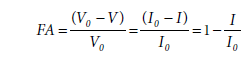where:
FA is the fractional absorbance.
V0 is the output in zero gas.
V is the output in target gas.

Rearranging and combining the previous two equations givesIf k and l are held constant, FA can be plotted against x as shown in Figure 6 (where kl = 115, 50, 25, 10, and 4.5). The value of FA increases with c, but eventually saturates at high gas concentrations.Figure 6. Typical Fractional Absorbance for kl = 4.5, 10, 25, 50, 115

This relationship implies that for any fixed setup, the ability to resolve a change in gas level is better at low concentrations than at high concentrations; however, k and l can be adjusted to provide the optimum absorbance for the required range of gas concentration. This means that long optical paths are more suited for low gas concentrations, and short optical paths are more suited for high gas concentrations.

The following describes a two-point calibration procedure necessary to determine the kl constant using the ideal Beer-Lambert equation. If b = kl, thenThe first part of the calibration requires applying low concentration CO2 gas (or pure nitrogen, which is 0% vol.) to the sensor assembly:

• ACTLOW is the peak-to-peak output of the active detector in low concentration gas.
• REFLOW is the peak-to-peak output of the reference detector in low concentration gas.
• TLOW is the temperature of the low concentration gas.

The second part of the calibration requires applying CO2 gas of a known concentration (xCAL) to the assembly. Usually, the xCAL concentration level is chosen to be the maximum value of the concentration range (for example, 0.5% vol. for the industrial air quality range).

• ACTCAL is the peak-to-peak output of the active detector in the calibration gas of concentration xCAL.
• REFCAL is the peak-to-peak output of the reference detector in the calibration gas of concentration xCAL.

The following two simultaneous equations in two unknowns (I0 and b) can then be written:Solving the two equations for I0 and b,Then, for a gas of unknown concentration (x), where

• ACT is the peak-to-peak output of the active detector in unknown gas.
• REF is the peak-to-peak output of the reference detector in unknown gas.
• T is the temperature of the unknown gas in K.The T/TLOW factor compensates for the change in concentration with temperature due to the ideal gas law.

Modified Beer-Lambert Law

Practical considerations in the NDIR implementation require modifications to the Beer-Lambert Law, as follows, to obtain accurate readings:The SPAN factor is introduced because not all the IR radiation that impinges upon the active thermopile is absorbed by the gas, even at high concentrations. SPAN is less than 1 because of the optical filter bandwidth and the fine structure of the in the absorption spectra.

Variations in the optical path length and light scattering require the addition of a power term, c, for accurately fitting the equation to the actual absorption data.

The value of the b and SPAN constants also depends upon the range of concentration measured. The typical concentration ranges are as follows:

• Industrial air quality (IAQ): 0 to 0.5% vol. (5000 ppm). Note that CO2 concentration in ambient air is approximately 0.04% vol., or 400 ppm.
• Safety: 0 to 5% vol.
• Combustion: 0 to 20% vol.
• Process control: 0 to 100% vol.

The exact values of b and c for a particular system are usually determined by taking a number of data points for FA vs. the concentration, x, and then using a curve fitting program.

For a given system where the b and c constants have been determined, the value of ZERO and SPAN can be calculated using the two- point calibration method.

The first step in the procedure it to apply a low gas concentration of xLOW and record the following:

• ACTLOW: the peak-to-peak output of the active detector in low concentration gas
• REFLOW: the peak-to-peak output of the reference detector in low concentration gas
• TLOW: the temperature of the low concentration gas in K.

The second part of the calibration requires applying CO2 gas of a known concentration (xCAL) to the assembly. Usually, the xCAL concentration level is chosen to be the maximum value of the concentration range (for example, 0.5% vol. for the industrial air quality range). Record the following:

• ACTCAL: the peak-to-peak output of active detector in the calibration gas of concentration xCAL.
• REFCAL: the peak-to-peak output of the reference detector in the calibration gas of concentration xCAL.

The following two simultaneous equations in two unknowns (I0 and SPAN) can then be written:Solving the two equations for ZERO and SPAN yieldsThen, for a gas of unknown concentration (x), where

• ACT is the peak-to-peak output of the active detector in unknown gas.
• REF is the peak-to-peak output of the reference detector in unknown gas.
• T is the temperature of the unknown gas in K.This equation assumes that TLOW = TCAL.

Effects of Ambient Temperature

The thermopile detector senses temperature by absorbing radiation, but it also responds to ambient temperature changes which can give rise to spurious and misleading signals. For this reason, many thermopiles have thermistors integrated into the package.

The radiation absorption is related to the number of target molecules in chamber, not the absolute percentage of target gas. Therefore the absorption is described by the ideal gas law at standard atmosphere pressure.

It is necessary to record the temperature data in both the calibration state and the measurement state: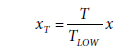where:
x is the concentration of gas without temperature compensation.
TLOW is the temperature in K at low and high gas concentration.
T is the temperature in K at sampling.
xT is the gas concentration at temperature T.

In addition to the ideal gas law variation of concentration with temperature, SPAN and FA vary slightly with temperature and may require correction for extremely high accuracy concentration measurements.

This circuit note does not deal with SPAN and FA temperature correction; however, details can be found in Application Note 1, Application Note 2, Application Note 3, Application Note 4, and Application Note 5 from SGX Sensortech, 2 Hanbury Road, Chelmsford, UK; and the AAN-201, AAN-202, AAN-203, AAN-204, and AAN-205 application notes from Alphasense Limited, Sensor Technology House, 300 Avenue West, Skyline 120, Great Notley Essex, UK.

Thermopile Driver

The HTS-E21-F3.91/F4.26 thermopile (Heimann Sensor, GmbH) has an 84 kΩ internal resistance in each channel. The equivalent circuit of the driver for one of the thermopile channels is shown in Figure 7. The internal 84 kΩ thermopile resistance and the external 8.2 nF capacitor form an RC low pass noise filter with a −3 dB cut off frequency:Changing C11 and C15 for various thermopiles also changes the noise performance and the response time.Figure 7. Thermopile Driver Equivalent Circuit, G = 214.6

The step function setting time of the 84 kΩ/8.2 nF filter to 22 bits is approximatelyThe AD8629 noninverting amplifier is set to a gain of 214.6 and the −3 dB cut off frequency:The settling time to 22 bits is approximately:The maximum NDIR chop frequency is 5 Hz, and the minimum half cycle pulse width is therefore 100 ms. The settling time to 22 bits is approximately 0.1 times the minimum chop pulse width.

The AD8629 has a 0.1 Hz to 10 Hz input voltage noise of 0.5 μV p-p. Ignoring the sensor voltage noise and the AD8629 current noise, a 1 mV p-p signal output from the thermopile yields a signal-to-noise ratio (SNR) of: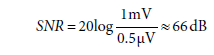One of thermopiles is connected as the pseudo differential input to the ADuCM360 ADC1/ADC3 pair, and the second is connected to the ADC2/ADC3 pair. The ADC3 input is connected to a common-mode voltage of 200 mV, driven by the ADA4528-1 low noise amplifier. The ADA4528-1 input 0.1 Hz to 10 Hz voltage noise is 99 nV p-p. The 200 mV common-mode voltage is required to keep the ADC input pins greater than 0.1 V.

The gain of the AD8629 stage is 214.6, and the gain of the internal PGA of ADuCM360 is automatically set by software from 1 to 128 to ensure the input signal matches the full-scale span of the ADC input, ±1.2 V. The peak-to-peak signal from the thermopile can range from a few hundred microvolts to several mV. For instance, if the full-scale thermopile signal is 1 mV p-p, a PGA gain of 4 produces an 860 mV p-p into the ADC.

Thermopiles with different sensitivities may require a different gain in the AD8629 stage. Interfacing the CN-0338 Arduino shield board with other Arduino-compatible platforms may require higher gains if the platform uses an ADC without an internal PGA.

The easiest way to change the AD8629 gain is to change R6 and R10; which does not affect the dominant pole frequency set by R5/R8 and C9/C10.

The thermopile output data processing algorithm can be selected in software. The user can select between peak-to-peak and averaging algorithms.

Further details regarding the acquisition of the signals, lamp pulse timing, along with the processing algorithms for temperature compensation are included in the CN-0338 source code found in the CN-0338 Design Support Package and in the CN-0338 User Guide.

NTC Thermistor Driver

The characteristics of the integrated NTC temperature sensor in thermopile are as follows:The Thevenin equivalent circuit for the thermistor driver is shown in Figure 8.The R3 and R4 divider resistors provide a 670.3 mV voltage source in series with the 103.6 kΩ. The driving voltage is 670.3 mV − 200 mV = 470.3 mV.Figure 8. NTC Thermistor Driver Equivalent Circuit

When RTH = 100 kΩ at 25°C, the voltage across the thermistor is 231 mV, and the PGA gain is therefore set at 4 when making the measurement.

The flexible input multiplexer and dual ADCs in the ADuCM360 allow simultaneous sampling of both the thermopile signals and the temperature sensor signal to compensate for drift.

IR Light Source Driver

The filament light source selected is the International Light Technologies MR3-1089, with polished aluminum reflector that requires a drive voltage of 5.0 V at 150 mA for maximum infrared emission and the best system performance. Heat from the lamp keeps the temperature of the optical reflector higher than ambient, which is helpful in preventing condensation in humid environments.

Filament lamps have a low resistance when cold (turned off), which can result in a current surge at the instant of turn-on. A regulator with a soft-start function is useful in addressing this problem. The ADP7105 low dropout voltage regulator has a programmable enable pin which can be used with a general-purpose input/output pin of the ADuCM360 to enable/disable the lamp voltage. A soft-start capacitor, C6, of 10 nF provides a soft-start time of 12.2 ms, which is approximately 0.125 times the minimum chop step time of 100 ms.

The lamp on-current (~150 mA) is large, therefore careful circuit design and layout is required to prevent the lamp switching pulses from coupling into the small thermopile output voltages.

Take care to ensure the lamp return path does not flow through the sensitive thermopile detector ground return path. The lamp current must not use the same return path as the processor; otherwise it may cause voltage offset errors. It is strongly recommended that a separate voltage regulator be used for the lamp drive and the signal-conditioning portion of the system.

The ADP7105 lamp driver is supplied directly from the external power supply connected to the EVAL-ADICUP360 board.

Software Considerations

Synchronized Chopping and Sampling

To measure the gas concentration, the peak-to-peak signal value in both the reference and active channel must be sampled. The ADuCM360 includes two 24-bit, Σ-Δ ADCs, and the ADCs operate in continuous sampling mode. Programmable gain amplifiers with gain options of 1, 2, 4, 8, 16, 32, 64, and 128 drive the ADCs.

The default chopping frequency is set to 0.25 Hz, and the default sampling rate is set to 10 Hz. However, the chopping frequency can be set in the software from 0.1 Hz to 5 Hz, and the ADC sampling rate from 3.5 Hz to 483 Hz. The software ensures that the sampling rate is at least 30 times the chopping frequency.

For the default chopping frequency of 0.25 Hz, the thermopile data is taken at a 10 Hz rate during the last 1.5 sec of the 2 sec half cycle to ensure that the signal has settled. The data during the first 500 ms is ignored (blanking time). The blanking time can also be set in the software, for both edges. Note that the NTC thermistor data is taken during the blanking time.

Calibration Procedure: Ideal Beer-Lambert Equation

Because of differences in the characteristics of lamps and thermopiles, the circuit must be calibrated initially and also after changing either the thermopile or the lamp.

It is recommended that the entire assembly be placed in a closed chamber where gas of known CO2 concentration can be injected until all existing gas in the chamber is flushed out. After stabilizing for a few minutes, the measurements can then be made.

The calibration method and algorithms are shown in the following steps for the ideal Beer-Lambert equation:

1. Input the following command: sbllcalibrate (standard Beer-Lambert calibration).
2. Inject low concentration, xLOW, or zero gas (nitrogen), and stabilize the chamber.
3. Input the CO2 concentration into the terminal.
4. The system measures ACTLOW, the peak-to-peak output of the active detector in low concentration gas.
5. The system measures REFLOW, the peak-to-peak output of the reference detector in low concentration gas.
6. The system measures temperature of low gas, TLOW.
7. Inject high concentration CO2, of concentration xCAL, into the chamber.
8. Input the CO2 concentration into the terminal.
9. The system measures ACTCAL, REFCAL, and the calibration temperature, TCAL.
10. The system calculates ZERO and b:To measure an unknown concentration of CO2 gas using the ideal Beer-Lambert equation, do the following:

1. Apply the unknown concentration of gas to the chamber and stabilize.
2. Measure ACT, the peak-to-peak output of the active detector.
3. Measure REF, the peak-to-peak output of the reference detector.
4. Measure the temperature, T, in Kelvin.
5. Use the ZERO value from the calibration.
6. Use the b value from the calibration
7. Calculate the fractional absorbance:1. Calculate the concentration and apply the ideal gas law temperature compensation:This procedure assumes that TLOW = TCAL.

Calibration Procedure: Modified Beer-Lambert Equation

If the constants b and c are known from measurements, use the following procedure.

1. Input the following command: mbllcalibrate (modified Beer-Lambert calibration).
2. Input the b and c constants.
3. Inject low concentration CO2 gas, xLOW (nitrogen), and stabilize the chamber.
4. Input the CO2 concentration into the terminal.
5. The system measures ACTLOW, the peak-to-peak output of the active detector in low gas.
6. The system measures REFLOW, the peak-to-peak output of the reference detector in low gas.
7. The system measures the temperature, TLOW.
8. Inject high concentration CO2, of concentration xCAL, into the chamber.
9. Input the CO2 concentration into the terminal.
10. The system measures ACTCAL, REFCAL, and the calibration temperature, TCAL.
11. The system calculates ZERO and SPAN:To measure an unknown concentration of CO2 gas using the modified Beer-Lambert equation, do the following:

1. Apply the unknown concentration of gas to the chamber and stabilize.
2. Measure ACT, the peak-to-peak output of the active detector.
3. Measure REF, the peak-to-peak output of the reference detector.
4. Measure the temperature, T, in kelvin.
5. Use the ZERO and SPAN values from calibration.
6. Use the values of b and c that were previously determined.
7. Calculate fractional absorbance: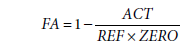1. Calculate the concentration and apply the ideal gas law temperature compensation:This procedure assumes that TLOW = TCAL.

NTC Thermistor Algorithm and Calculations

The NTC thermistor equivalent circuit is shown in Figure 9.Figure 9. NTC Thermistor Circuit

The voltage across the thermistor is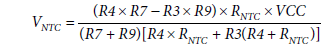where:
VCC is 3.3 V.
RNTC is thermistor resistance.

The NTC thermistor resistance can be expressed aswhere:
RTH is the thermistor resistance at temperature T0.
β is a parameter given in the NTC thermistor data sheet.
RNTC is the the thermistor resistance at temperature T.

Combining the two equations yields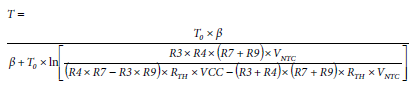During each lamp chopping time interval, the ADC is switched to NTC sampling, as shown in Figure 10.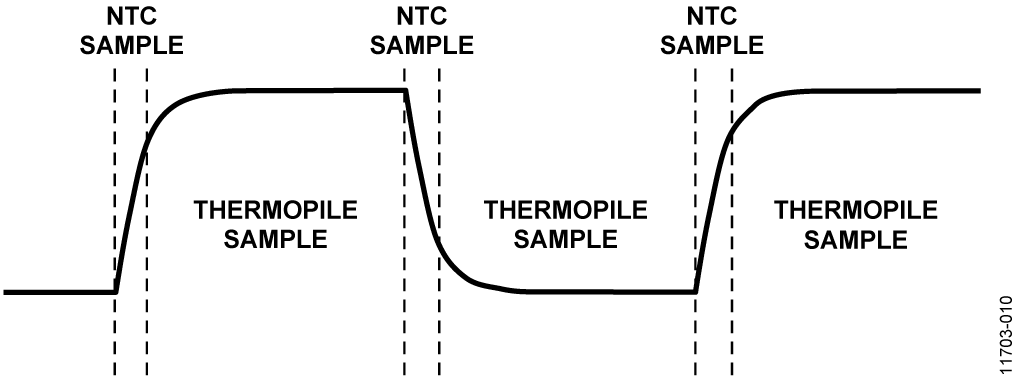Figure 10. Timing of NTC and Thermopile Sampling and Lamp Chopping

Human Interactive Interface

The EVAL-ADICUP360 platform board connects to the PC via the USB port. The board appears as a virtual COM device, and the default COM port setting is 115200 Hz, 1 start bit, 8 data bits, no parity bits, and 2 stop bits. The circuit does not respond if the baud rate setting is incorrect. The user has the ability to reset the baud rate to the default value by pressing the RESET button on the EVAL-ADICUP360 board. Note that performing an EVAL-ADICUP360 reset only resets the baud rate value; other CN-0338 settings keep their values. To reset all these parameters to default, use the resetTodefault command.

Any type of serial port terminal can be used to interact with the EVAL-ADICUP360 board for development and debugging. Set the terminal or no echo typed characters locally, because the EVAL-ADICUP360 echoes back each character from the PC.

The CN-0338 software use the P0.1 and P0.2 port of the ADuCM360 as the UART communicate pins. Set the EVAL-ADICUP360 jumper switch according to which USB port is connected to the PC. See the EVAL-ADICUP360 product page for more details.

After connecting successfully, follow the terminal directions to operate the EVAL-CN0338-ARDZ board.

Figure 11 shows the fractional absorbance (FA) as a function of CO2 concentration for a typical EVAL-CN0338-ARDZ board.Figure 11. Fractional Absorbance vs. CO2 Concentration for Typical CN-0338 Board

A complete design support package for the EVAL-CN0338-ARDZ board including layouts, bill of materials, schematics, and source code can be found at www.analog.com/CN0338-DesignSupport.

# Common Variations

The EVAL-CN0338-ARDZ board is an Arduino shield board and can transfer to other Arduino-compatible platforms. However, if the target Arduino-compatible platform does not have high precision ADCs on the board, the user must adjust the gain of the AD8629 to fit the signal to the ADC by changing R6 and R10. The common-mode voltage can also be changed by setting R7 and R9.

# Circuit Evaluation & Test

This circuit uses the EVAL-CN0338-ARDZ shield circuit board, an external power supply, PC computer with serial port terminal program, and the EVAL-ADICUP360 Arduino-compatible platform board. A user guide for the platform board can be found at www.analog.com/EVAL-ADICUP360_User_Guide.

A user guide for the EVAL-CN0338-ARDZ board can be found at www.analog.com/CN0338-UserGuide.

Equipment Needed

The following equipment is needed:

• EVAL-CN0338-ARDZ circuit board.
• PC with a USB port and 64-bit Linux distribution OS kernel v4.2.0-amd64 or later, with the terminal program.
• 7 V to 12 V/1 A dc power supply, or equivalent 7 V to 12 V/1 A bench supply.
• Calibration gas with 0 ppm to 100 ppm CO2 concentration (for example, nitrogen).
• Maximum concentration calibration gas with 5,000 ppm (0.5% vol.) CO2 concentration. Other values can be used for maximum concentration depending on the application.

Test Setup Functional Block Diagram

A functional diagram of the test setup is shown in Figure 12.Figure 12. Test Setup Functional Block Diagram

Setup

Take the following steps to set up the circuit for evaluation:

1. Plug the EVAL-CN0338-ARDZ shield board into the EVAL-ADICUP360 platform board.
2. Following the instructions in the EVAL-ADICUP360 user guide, connect the EVAL-ADICUP360 virtual COM USB port to the PC.
3. Following the instructions in the EVAL-ADICUP360 user guide, power on the EVAL-ADICUP360 with a 7 V to 12 V/1 A dc power supply.
4. Set up the terminal software using the 115200 Hz baud rate and the correct virtual COM port.
5. If the system does not have the correct baud rate setting, set the default value by pressing the RESET button on the EVAL-ADICUP360 board.
6. Following the prompt to start the CN-0338, perform the system calibration routine for first time use or hardware change.
7. The calibration routine uses the standard (ideal) Beer-Lambert law for the sbllcalibrate command and the modified Beer-Lambert law for the mbllcalibrate command. The user must enter the b and c constants when using the modified Beer-Lambert law.
8. Apply low concentration gas and input the concentration in percentage, xLOW.
9. Apply maximum concentration calibration gas and input the concentration level, xCAL. The program calculates the required coefficients. The constants are stored in nonvolatile memory until another calibration routine is performed.
10. If the calibration routine is bypassed, default values for ZERO, b, and TLOW are used in the ideal Beer-Lambert equation computation.
11. Input the run command, and the program now continuously displays the concentration level of CO2. See the CN-0338 User Guide for further details.

A photograph of the EVAL-CN0338-ARDZ Arduino shield board is shown in Figure 13.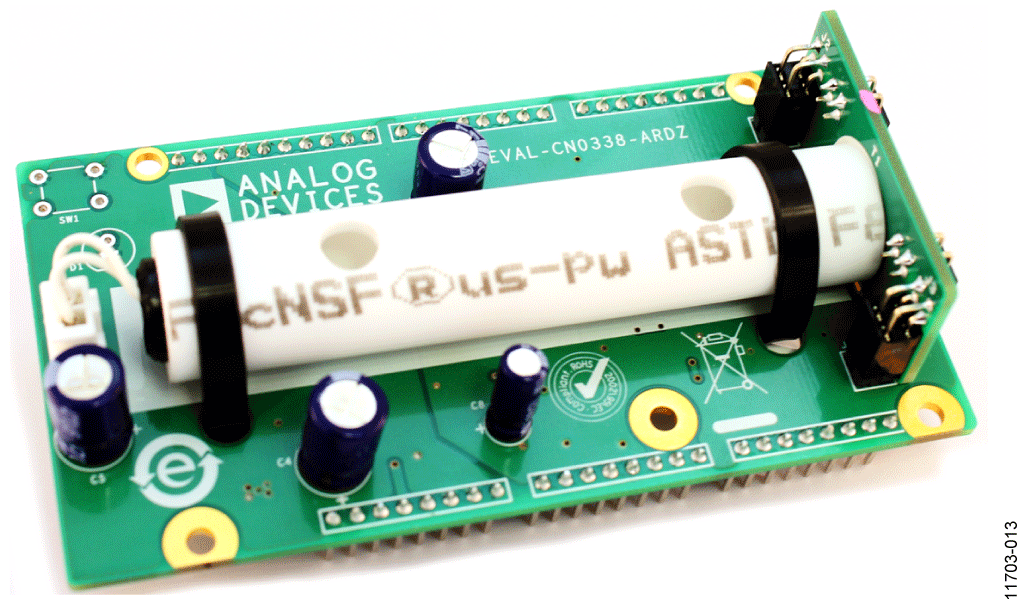Figure 13. EVAL-CN0338-ARDZ Board Photo